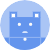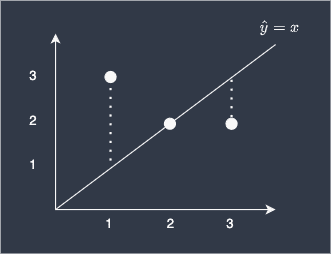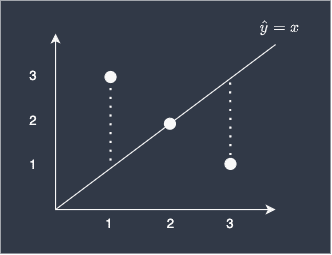search
Search
menu search toc more_vert
Guest 0reps
Thanks for the thanks!
close
chevron_left Model performance
Cancel
Post
account_circle
Profile
exit_to_app
Sign out
help Ask a question
search
keyboard_voice
close
Searching Tips
Search for a recipe:
"Creating a table in MySQL"
Search for an API documentation: "@append"
Search for code: "!dataframe"
Apply a tag filter: "#python"
Useful Shortcuts
/ to open search panel
Esc to close search panel
to navigate between search results
d to clear all current filters
Enter to expand content previewDoc SearchCode Search BetaSORRY NOTHING FOUND!
mic
Start speaking...Voice search is only supported in Safari and Chrome.
Shrink
Navigate to
A
A
brightness_medium
share
arrow_backShareTwitterFacebook
chevron_left Model performance
1
thumb_down
0
chat_bubble_outline
0
auto_stories new
settings

# Comprehensive Guide on Mean Squared Error (MSE)

Machine Learning
chevron_right
Model performance
schedule Jul 1, 2022
Last updated
local_offer
Tags

# What is mean squared error (MSE)?

The mean squared error, or MSE, is a performance metric that indicates how well your model fits the target. The mean squared error is defined as the average of all squared differences between the true and predicted values:

$$\mathrm{MSE}=\frac{1}{n}\sum^{n-1}_{i=0}(y_i-\hat{y}_i)^2$$

Where:

• $n$ is the number of predicted values

• $y_i$ is the actual true value of the $i$-th data

• $\hat{y}_i$ is the predicted value of the $i$-th data

A high value of MSE means that the model is not performing well, whereas a MSE of 0 would mean that you have a perfect model that predicts the target without any error.

# Simple example of computing mean squared error (MSE)

Suppose we are given the three data points (1,3), (2,2) and (3,2). To predict the y-value given the x-value, we've built a simple learn curve, $y=x$, as shown below:We can see that we are off by 2 for the first data point, the prediction is perfect for the second point, and off by 1 for the last point.

To quantify how good our model is, we can compute the MSE like so:

\begin{align*} \mathrm{MSE}&=\frac{1}{3}\left[(1-3)^2+(2-2)^2+(3-2)^2\right]\\ &=\frac{1}{3}\left(4+0+1\right)\\ &\approx1.67 \end{align*}

This means that the average squared differences between the true value and the predicted value is 1.67.

# Intuition behind mean squared error (MSE)

## Interpretation of MSE

MSE is defined as the average squared differences between the actual values and the predicted values. This makes the interpretation of MSE rather awkward since the unit of MSE is not the same as the unit of the y-values due to squaring the differences. Therefore, we typically interpret a high value of MSE as indicative of a poor-performing model, while a low value of MSE as indicative of a decent model.

There is another performance metric called root mean squared error (RMSE), which is simply the square root of MSE. This means that the RMSE takes on the same unit as that of the target values, which implies you can loosely interpret RMSE as the average difference between the actual and predicted values.

## Why are we squaring the difference?

The reason we take the square when calculating MSE is that we care only about the magnitude of the differences between true and predicted value - we do not want the positive and negative differences cancelling each other out. For example, consider the following case:Suppose we computed the MSE without taking the square:

$$\frac{1}{3}\left[(1-3)+(2-2)+(3-1)\right]=0$$

You can see that the negative difference and the positive difference of the first and third data points cancel each other out, resulting in a misleading error benchmark of 0. Of course, we know that the model is far from perfect in reality. In order to avoid such problems, we square the differences.

## Why don't we just take the absolute difference instead?

You may be wondering why we don't just take the absolute difference between the true and predicted value if all we care about is the magnitude of the differences. In fact, there is another popular metric called mean absolute error (MAE) that does just this. The advantage of absolute mean error is that the interpretation is simple - the error is just how off your predictions are from the true value on average.

The caveat, however, is that it is not easy to find minimum values of MAE, which means that it is challenging to train a model that minimises MAE. On the other hand, MSE is easily differentiable and hence easy to optimise. This is reason why MSE is preferred over MAE as the cost function of machine learning models.

# Computing the mean squared error (MSE) in Python's Scikit-learn

Let's compute the MSE for the example above using Python's scikit-learn library. To compute the MSE in scikit-learn, simply use the mean_squared_error method:

 from sklearn.metrics import mean_squared_errory_true = [1,2,3]y_pred = [3,2,2]mean_squared_error(y_true, y_pred) 1.6666666666666667 

We can see that the outputted MSE is exactly the same as the value we manually calculated above.

## Setting multioutput

By default, multioutput='uniform_average', which returns a the global mean squared error:

 y_true = [[1,2],[3,4]]y_pred = [[6,7],[9,8]]mean_squared_error(y_true, y_pred) 25.5 

Setting multioutput='raw_values' will return mean squared error of each column:

 y_true = [[1,2],[3,4]]y_pred = [[6,7],[9,8]]mean_squared_error(y_true, y_pred, multioutput='raw_values') array([30.5, 20.5]) 

Here, 30.5 is calculated as:

 ((1-6)^2 + (3-9)^2) / 2 = 30.5 
mail